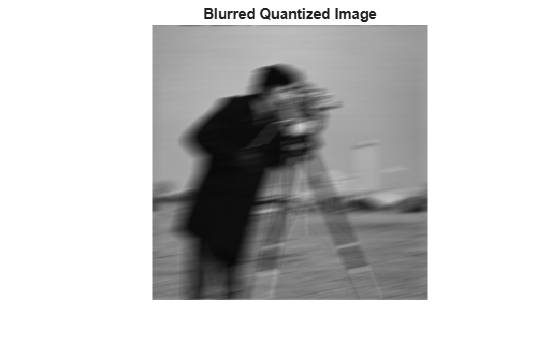## Deblur Images Using a Wiener Filter

This example shows how to use Wiener deconvolution to deblur images. Wiener deconvolution can be used effectively when the frequency characteristics of the image and additive noise are known, to at least some degree.

Read and display a pristine image that does not have blur or noise.

```Ioriginal = imread('cameraman.tif'); imshow(Ioriginal) title('Original Image')```### Simulate and Restore Motion Blur Without Noise

Simulate a blurred image that might result from camera motion. First, create a point-spread function, `PSF`, by using the `fspecial` function and specifying linear motion across 21 pixels at an angle of 11 degrees. Then, convolve the point-spread function with the image by using `imfilter`.

The original image has data type `uint8`. If you pass a `uint8` image to `imfilter`, then the function will quantize the output in order to return another `uint8` image. To reduce quantization errors, convert the image to `double` before calling `imfilter`.

```PSF = fspecial('motion',21,11); Idouble = im2double(Ioriginal); blurred = imfilter(Idouble,PSF,'conv','circular'); imshow(blurred) title('Blurred Image')```Restore the blurred image by using the `deconvwnr` function. The blurred image does not have noise so you can omit the noise-to-signal (NSR) input argument.

```wnr1 = deconvwnr(blurred,PSF); imshow(wnr1) title('Restored Blurred Image')```### Simulate and Restore Motion Blur and Gaussian Noise

Add zero-mean Gaussian noise to the blurred image by using the `imnoise` function.

```noise_mean = 0; noise_var = 0.0001; blurred_noisy = imnoise(blurred,'gaussian',noise_mean,noise_var); imshow(blurred_noisy) title('Blurred and Noisy Image')```Try to restore the blurred noisy image by using `deconvwnr` without providing a noise estimate. By default, the Wiener restoration filter assumes the NSR is equal to 0. In this case, the Wiener restoration filter is equivalent to an ideal inverse filter, which can be extremely sensitive to noise in the input image.

In this example, the noise in this restoration is amplified to such a degree that the image content is lost.

```wnr2 = deconvwnr(blurred_noisy,PSF); imshow(wnr2) title('Restoration of Blurred Noisy Image (NSR = 0)')```Try to restore the blurred noisy image by using `deconvwnr` with a more realistic value of the estimated noise.

```signal_var = var(Idouble(:)); NSR = noise_var / signal_var; wnr3 = deconvwnr(blurred_noisy,PSF,NSR); imshow(wnr3) title('Restoration of Blurred Noisy Image (Estimated NSR)')```### Simulate and Restore Motion Blur and 8-Bit Quantization Noise

Even a visually imperceptible amount of noise can affect the result. One source of noise is quantization errors from working with images in `uint8` representation. Earlier, to avoid quantization errors, this example simulated a blurred image from a pristine image in data type `double`. Now, to explore the impact of quantization errors on the restoration, simulate a blurred image from the pristine image in the original `uint8` data type.

```blurred_quantized = imfilter(Ioriginal,PSF,'conv','circular'); imshow(blurred_quantized) title('Blurred Quantized Image')```Try to restore the blurred quantized image by using `deconvwnr` without providing a noise estimate. Even though no additional noise was added, this restoration is degraded compared to the restoration of the blurred image in data type `double`.

```wnr4 = deconvwnr(blurred_quantized,PSF); imshow(wnr4) title('Restoration of Blurred Quantized Image (NSR = 0)');```Try to restore the blurred quantized image by using `deconvwnr` with a more realistic value of the estimated noise.

```uniform_quantization_var = (1/256)^2 / 12; signal_var = var(Idouble(:)); NSR = uniform_quantization_var / signal_var; wnr5 = deconvwnr(blurred_quantized,PSF,NSR); imshow(wnr5) title('Restoration of Blurred Quantized Image (Estimated NSR)');```